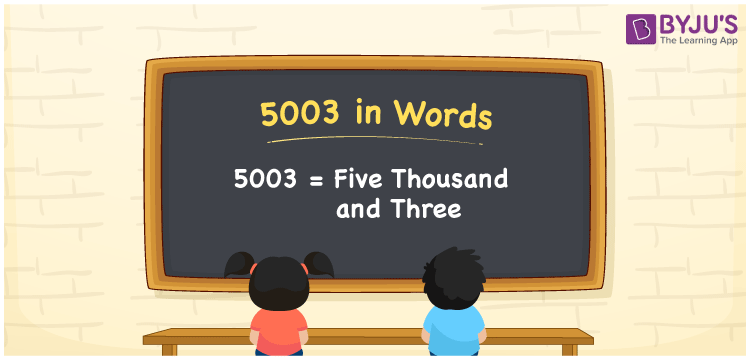# 5003 in words

5003 in words is written as Five Thousand and Three. 5003 represents the count or value. The article on Counting Numbers can give you an idea about count or counting. The number 5003 is used in expressions that relate to money, distance, length, year and others. Let us consider an example for 5003. ”The cash presentation I received for my birthday party was Five Thousand and Three rupees.”

 5003 in words Five Thousand and Three Five Thousand and Three in Numbers 5003

## 5003 in English Words## How to Write 5003 in Words?

We can convert 5003 to words using a place value chart. The number 5003 has 4 digits, so let’s make a chart that shows the place value up to 4 digits.

 Thousands Hundreds Tens Ones 5 0 0 3

Thus, we can write the expanded form as:

5 × Thousand + 0 × Hundred + 0 × Ten + 3 × One

= 5 × 1000 + 0 × 100 + 0 × 10 + 3 × 1

= 5003

= Five Thousand and Three.

5003 is the natural number that is succeeded by 5002 and preceded by 5004.

5003 in words – Five Thousand and Three.

Is 5003 an odd number? – Yes.

Is 5003 an even number? – No.

Is 5003 a perfect square number? – No.

Is 5003 a perfect cube number? – No.

Is 5003 a prime number? – Yes.

Is 5003 a composite number? – No.

## Solved Example

1. Write the number 5003 in expanded form

Solution: 5 × 1000 + 0 × 100 + 0 × 10 + 3 × 1

We can write 5003 = 5000 + 000 + 00 + 3

= 5 × 1000 + 0 × 100 + 0 × 10 + 3 × 1

## Frequently Asked Questions on 5003 in words

### How to write 5003 in words?

5003 in words is written as Five Thousand and Three.

### Is 5003 a perfect square number?

No. 5003 is not a perfect square number.

### Is 5003 a prime number?

Yes. 5003 is a prime number.